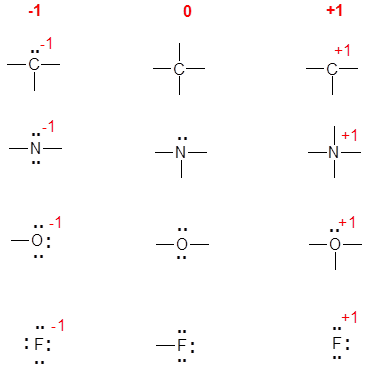# Formal Charges

$$\newcommand{\vecs}{\overset { \rightharpoonup} {\mathbf{#1}} }$$ $$\newcommand{\vecd}{\overset{-\!-\!\rightharpoonup}{\vphantom{a}\smash {#1}}}$$$$\newcommand{\id}{\mathrm{id}}$$ $$\newcommand{\Span}{\mathrm{span}}$$ $$\newcommand{\kernel}{\mathrm{null}\,}$$ $$\newcommand{\range}{\mathrm{range}\,}$$ $$\newcommand{\RealPart}{\mathrm{Re}}$$ $$\newcommand{\ImaginaryPart}{\mathrm{Im}}$$ $$\newcommand{\Argument}{\mathrm{Arg}}$$ $$\newcommand{\norm}{\| #1 \|}$$ $$\newcommand{\inner}{\langle #1, #2 \rangle}$$ $$\newcommand{\Span}{\mathrm{span}}$$ $$\newcommand{\id}{\mathrm{id}}$$ $$\newcommand{\Span}{\mathrm{span}}$$ $$\newcommand{\kernel}{\mathrm{null}\,}$$ $$\newcommand{\range}{\mathrm{range}\,}$$ $$\newcommand{\RealPart}{\mathrm{Re}}$$ $$\newcommand{\ImaginaryPart}{\mathrm{Im}}$$ $$\newcommand{\Argument}{\mathrm{Arg}}$$ $$\newcommand{\norm}{\| #1 \|}$$ $$\newcommand{\inner}{\langle #1, #2 \rangle}$$ $$\newcommand{\Span}{\mathrm{span}}$$$$\newcommand{\AA}{\unicode[.8,0]{x212B}}$$

##### Key Points
• Not all atoms within a neutral molecule have to be neutral.
• Atoms within a molecule with formal charges are often the site of chemical reactions.
• You should be able to determine the formal charge for any atom in any molecule.

Knowing the formal charges on specific atoms in a molecule is an important step in keeping tract of the electrons and determine the chemical reactivity of the molecule. Formal charges can be calculated mathematically, but they can also be determined by intuition. The instinctive method is faster but requires more skill and knowledge of common structures.

## Mathematical Method

Formal charge equation is based on the comparing the number of electrons in the individual atom with that in the structure. For each atom, we then compute a formal charge:

$\text{formal charge}= \underbrace{valence\; e^-}_{free\; atom } - \underbrace{ \left ( nonbonding\; e^{-}+\dfrac{bonding\;e^{-}}{2} \right ) }_{atom\; in\; Lewis\; structure } \label{8.5.1}$

where the group number is equal to the number of electrons of the neutral atom.

## Intuitive Method

The instinctive method relies on experience and the understanding of common, known neutral structures. To do this you need to know the common bonding patterns for C, N, O, and the halides:

• C: 4 bonds;
• N: 3 bonds, 1 lone pair;
• O: 2 bonds, 2 lone pairs;
• F: 1 bond, 3 lone pairs (or any other halide).

Once mastered, this is much easier and quicker. The diagram below shows the common bonding situations for C, N, O, and F. The central column is the neutral atom, in the left most column the atom has gained an electron by having a bonding pair converted to a lone pair of electrons. The right most column has a formal charge of +1 due to either: a) using a lone pair of electrons as a bonding pair (N and O), b) losing a bonding pair of electrons (C), or c) losing a lone pair of electrons (F).Formal Charges is shared under a CC BY-NC-SA 4.0 license and was authored, remixed, and/or curated by Richard Spinney.